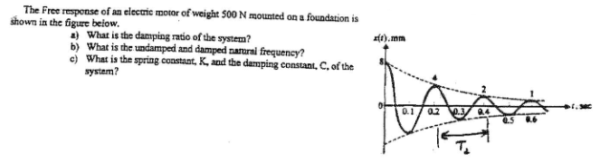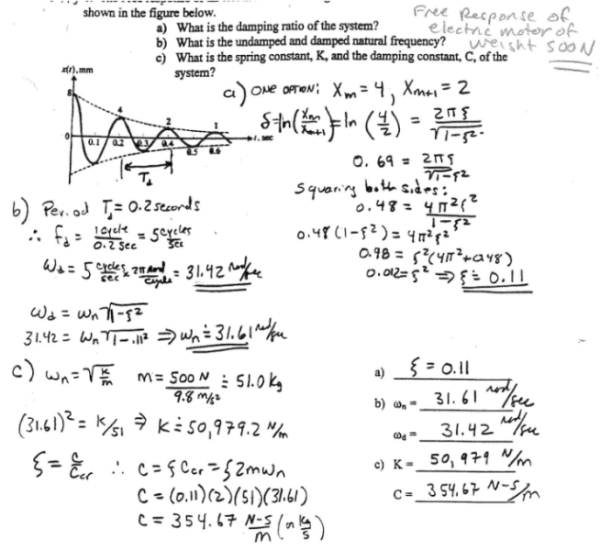The free response of an electric motor of weight 500 N mounted on a foundation is shown in the figure below. a) What is the damping ratio of the system? b) What is the undamped and damped natural frequency? c) What is the spring constant, K, and the damping constant, C, of the system?The free response of an electric motor of weight 500 N mounted on a foundation is shown in the figure below. a) What is the damping ratio of the system? b) What is the undamped and damped natural frequency? c) What is the spring constant, K, and the damping constant, C, of the system?

Vibrations Page 1 vibrations vibrations vibrations vibrations vibrations vibrations vibrations Vibrations Page 2